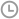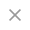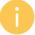Excel一般常用的查找函数

•发布时间：2018-10-12 06:46:18
• |
• 出处：PDF猫
• |
• 阅读量：653
Excel一般常用的查找函数就属于vlookup函数、lookup函数、index函数是最常用的查询函数，既方便快捷而且准确性高。

1、简单查找
=VLOOKUP(E2,A:C,3,0)2、多条件查找
=INDEX(A:D,MATCH(F2,A:A,0),MATCH(G2,1:1,0))名查找总业绩3、查找最后一个符合条件的值
=LOOKUP(2,1/(A2:A9=A12),E2:E9)PS：多条件查找最后符合条件的值
=LOOKUP(2,1/((A2:A9=A12)*(D2:D9=D12)),E2:E9)4、根据区间取数
{=LOOKUP(D9,IF(B\$2:B\$7=B9,D\$2:D\$7),C\$2:C\$7)}PDF猫转换器

PDF猫转换器